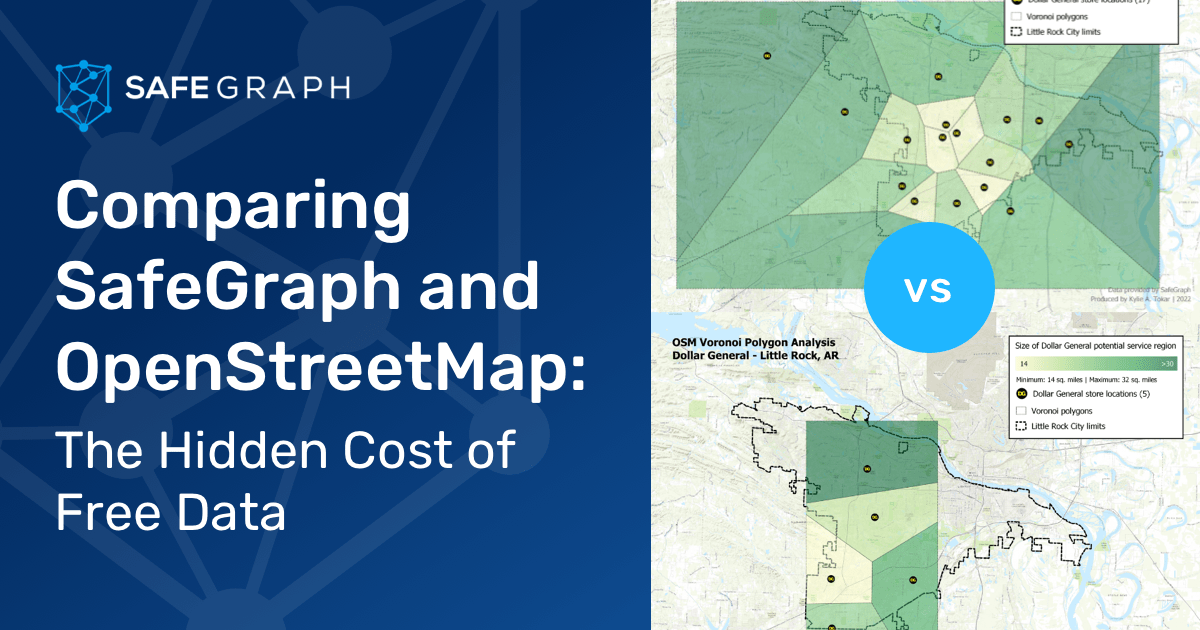Blog home

# A Non-Technical Introduction to Machine Learning

March 3, 2017
by
Noah Yonack

## Why should I read this, and what will I learn?

Machine learning is a field that threatens to both augment and undermine exactly what it means to be human, and it’s becoming increasingly important that you—yes, you—actually understand it.

I don’t think you should need to have a technical background to know what machine learning is or how it’s done. Too much of the discussion about this field is either too technical or too uninformed, and, through this blog, I hope to level the playing field.

This is for smart, ambitious people who want to know more about machine learning but who don’t care about the esoteric statistical and computational details underlying the field. You don’t need to know any math, statistics, or computer science to read and understand it.

By the end of this post, you’ll:

1. Understand the basic logical framework of machine learning (ML).
2. Be able to define important relevant terms and concepts that anyone interested in this field should know. These terms are highlighted in boldface.
3. Know which high-level decisions go into building statistical models, and understand some of the implications of these decisions.
4. Be able to better analyze the question of when we should use the results of ML to make big decisions, such as determining public policy.

This overview is in no way comprehensive. Huge portions of the field are left out, either because they are too rare to merit study by non-technical decision makers, because they’re difficult to explain, or both.

## What is machine learning?

The field itself: ML is a field of study which harnesses principles of computer science and statistics to create statistical models. These models are generally used to do two things:

1. Prediction: make predictions about the future based on data about the past
2. Inference: discover patterns in data

Difference between ML and AI: There is no universally agreed upon distinction between ML and artificial intelligence (AI). AI usually concentrates on programming computers to make decisions (based on ML models and sets of logical rules), whereas ML focuses more on making predictions about the future.

They are highly interconnected fields, and, for most non-technical purposes, they are the same.

## What’s a statistical model?

Models: Teaching a computer to make predictions involves feeding data into machine learning models, which are representations of how the world supposedly works. If I tell a statistical model that the world works a certain way (say, for example, that taller people make more money than shorter people), then this model can then tell me who it thinks will make more money, between Cathy, who is 5’2”, and Jill, who is 5’9”.

What does a model actually look like? Surely the concept of a model makes sense in the abstract, but knowing this is just half the battle. You should also know how it’s represented inside of a computer, or what it would look like if you wrote it down on paper.

A model is just a mathematical function, which is merely a relationship between a set of inputs and a set of outputs. Here’s an example:

f(x) = x²

This is a function that takes as input a number and returns that number squared. So, f(1) = 1, f(2) = 4, f(3) = 9.

Let’s briefly return to the example of the model that predicts income from height. I may believe, based on what I’ve seen in the corporate world, that a given human’s annual income is, on average, equal to her height (in inches) times 1,000. So, if you’re 60 inches tall (5 feet), then I’ll guess that you probably make \$60,000 a year. If you’re a foot taller, I think you’ll make \$72,000 a year.

This model can be represented mathematically as follows:

Income = Height × \$1,000

In other words, income is a function of height.

Here’s the main point: Machine learning refers to a set of techniques for estimating functions (like the one involving income) based on datasets (pairs of heights and their associated incomes). These functions, which are called models, can then be used for predictions of future data.

Algorithms: These functions are estimated using algorithms. In this context, an algorithm is a predefined set of steps that takes as input a bunch of data and then transforms it through mathematical operations. You can think of an algorithm like a recipe — first do this, then do that, then do this. Done.

Machine learning of all types uses models and algorithms as its building blocks to make predictions and inferences about the world.

Now I’ll show you how models actually work by breaking them apart, component by component. This next part is important.

## A framework for understanding ML:

Inputs: Statistical models learn from the past, formatted as structured tables of data (called training data). These datasets — such as those you might find in Excel sheets — tend to be formatted in a very structured, easy-to-understand way: each row in the dataset represents an individual observation, also called a datum or measurement, and each column represents a different feature, also called a predictor, of an observation.

For example, you might imagine a dataset about people, in which each row represents a different person, and each column represents a different feature about that person: height, weight, age, income, etc.

Most traditional models accept data formatted in the way I’ve just described. We call this structured data.

Because one common goal of ML is to make predictions (for example, about someone’s income), training data also includes a column containing the data you want to predict. This feature is called the response variable (or output variable, or dependent variable) and looks just like any other feature in the table.

Most common statistical models are constructed using a technique called supervised learning, which uses data that includes a response variable to make predictions or do inference. There is also a branch of ML called unsupervised learning, which doesn’t require a response variable and which is generally used just to find interesting patterns between variables (this pattern-finding process is known as inference). It is just as important as supervised learning, but it is usually much harder to understand and also less common in practice. This document won’t talk much about the latter subfield. The takeaway from this paragraph is simply that there are two “types” of learning, and that supervised learning is more common.

Continuing the people dataset example, we might try to predict someone’s income based on her name, age, and height, so our training dataset would look like this: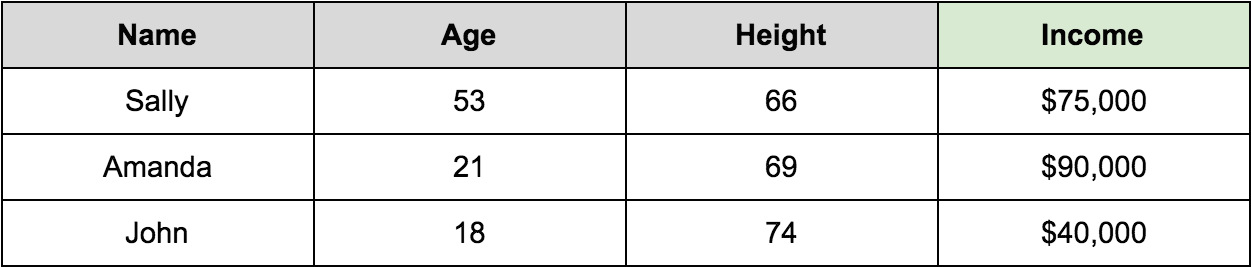Example of structured data. Each row is a person (a unique observation we’ve made), and each column is a different measurement (called a feature or predictor) of that person.

Is this problem suitable for prediction? Now that we have a dataset, we can begin building a statistical model.

Why should we do this? Well, we assume that there’s some relationship between our predictors and our response — that income is somehow based on your age and your height, or a combination of the two. It’s reasonable to assume that you make more money as you get older, for instance, and that your height subtly influences your job prospects.

We’ll leave the task of figuring out the exact details of the relationship (for instance, the precise role that age plays in determining your income) to the machine learning algorithm.

Model selection: We have our data, and we’ve decided that there’s probably a relationship between our predictors and our response. We’re ready to make predictions.

As an aside, we don’t actually need to know if there’s a relationship between these variables. We could, in fact, just throw all of our data into an algorithm and see if the resulting model is able to make valid predictions.

Now we need to pick which model to use. Naturally, there are many different types of models which explain how the data actually works, and we’d like to choose the one that most accurately describes the relationship between the predictors and the response variable.

Models generally fall into one of two categories:

1. Regression models, which are used when the response variable (i.e. the variable that you’re predicting) is continuous. For example, height, age, and income are all continuous. That is, they can be placed and ordered on a number line.
2. Classification models, which are used for categorical data — that is, data that doesn’t have a numerical ordering. For example, you may want to predict, based on an image of a flower, the species of that flower. Or you may want to predict whether a student is a psychology major or a math major.

The first step in picking a model is deciding whether or not your response variable is quantitative or categorical.

Putting on the brakes: Why is model selection an important concept for non-technical people? Well, if a model is chosen poorly, then its predictions will be inaccurate, leading to tangible actions and policies that are uninformed. As a high-level decision maker, it’s important for you to question why certain models are being employed so that you don’t end up making even poorer decisions down the road.

Below, I’ll walk you through an example of a popular, powerful, simple model from each category that can be used for prediction.

## A common regression model: simple linear regression

Simple linear regression: Chances are, you’ve constructed many simple linear regression models in the past. Again, a model is just a mathematical function, which describes a relationship between an input and an output.

A (simple) linear regression model is no different. We can describe the general case as follows:

y = m × X + b

The equation above relates the y value of a point to its X value. Specifically, if we know the X value, we can get the y value by multiplying X by m (called the slope) and then adding b (called the y intercept).

If you think that there exists a linear relationship between your features and your response — between, say, height and income — then you might be inclined to use a linear regression model. In other words, this model is a good choice if you think you can describe the relationship between height and income with a straight line.

So, let’s take this from the start. You find yourself with some data…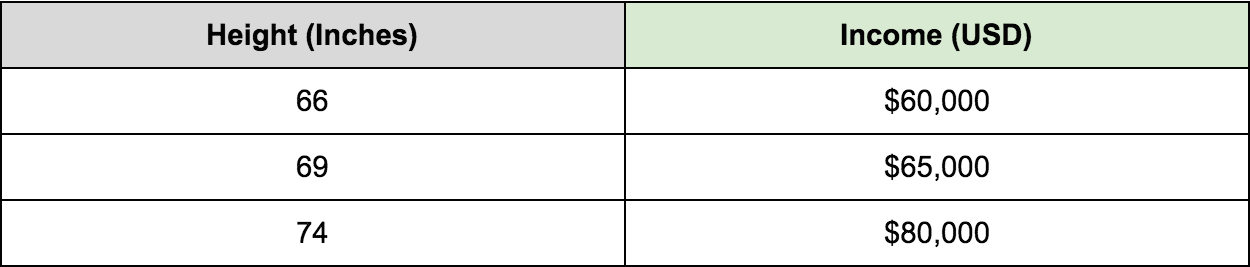Structured training data for our simple linear regression model.

…and you decide that you’d like to build a machine learning model to predict someone’s income based on their height.

First, you’d plot the two variables on an XY-plane to visually observe the relationship. Perhaps you’d see something like this: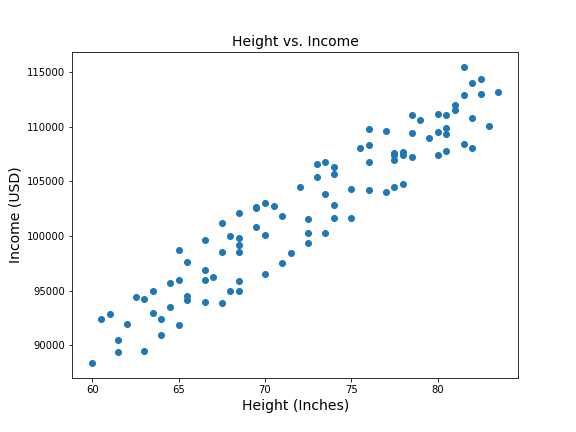A scatterplot of height vs. annual income. Each blue dot is an individual observation.

It looks like drawing a straight line somewhere through the blue dots would accurately characterize the visual relationship we’re seeing. This straight line, of course, can be described by the function I wrote out above:

y = m × X + b

Now I’d like to build a linear regression model. To do this, I train my model on my dataset: the linear regression algorithm will estimate the values of m and b, giving us an equation for a line that we can then use for prediction.

Putting on the brakes: We know that a model learns by looking at the training data and applying an algorithm. But what can go wrong if the training data is unrepresentative, biased, or incorrect?

Models don’t know whether or not training data is “good” — that’s up the human who trains the model. Unrepresentative training data can cause biased predictions which perpetuate social inequality.

For example, imagine a situation in which a model used in the criminal justice system is trained on data which is biased against African Americans. This model’s predictions, unsurprisingly, will then mirror that bias.

The details of the linear regression algorithm aren’t totally important, but you should know that the algorithm isn’t random. It takes your training data, applies some fancy linear algebra to it, and comes back with the “best” possible line.

Here’s what that line might look like: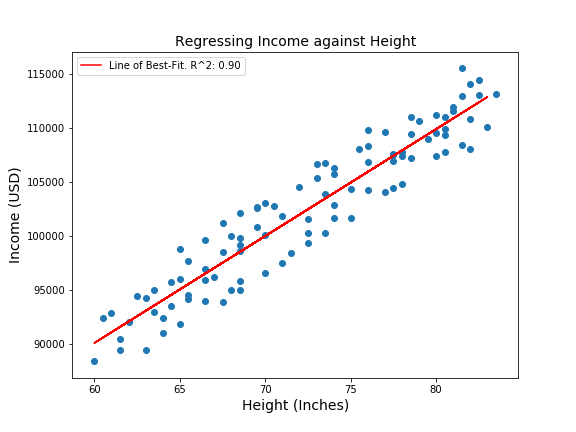The linear regression line (red) plotted against the true data (blue)

The linear regression model, then, can be written as follows:

Income = \$989 × Height + \$30,687

This model implies that for every inch you grow, you can expect to make \$989 more annually.

Putting on the brakes: It’s unbelievably important to interpret these model parameters correctly. What we’ve found here is a correlation between height and income, not a causation. Being taller doesn’t directly cause your income to increase, of course. Rather, being tall usually means that people treat you with more respect (at least in Western culture), which can give you a leg up in job interviews and salary negotiations, thereby increasing your income.

This example teaches us a valuable lesson about ML: it’s not magic. It won’t discover fundamental truths about the world around us. Rather, it will tell us how certain variables — like height and income — correlate with one another. Concluding from the model above that height causes increased income is an incorrect conclusion, and failing to understand that these patterns are merely correlational might cause you to make inappropriate business or policy decisions down the road.

We now know enough to truly understand what the learning in machine learning means. Many models, including linear regression, have parameters — which are variables (e.g. the m and the b in y = m × X + b) that the algorithm estimates (read: learns) by examining the training data. This process is called training.

To drive this point home, the simple linear model used above has two parameters that needed learning: m and b. By running the linear regression algorithm, the model estimated m to be \$989 and b to be \$30,687.

Evaluation function: How do we know that this line is best? This is a subtle question, and there’s no universally agreed upon way to answer this question.

Best, of course, is a relative term, and, in this case, we’ve defined the best line to be the one that maximizes R² on the testing data (pronounced R-squared, also called the coefficient of determination), which we can calculate by applying an evaluation function to our results.

An evaluation function is merely a way of determining just how good our predictions were. The way this is calculated in practice is to train a model on training data, but to reserve a handful of data (called testing data) for evaluation purposes.

Once your model is trained, you can hide the response variable in the testing set and run the rest of the test data through your model, resulting in a series of predictions. Then, you can compare your predictions to the true values of the response variable in the testing set, and use the evaluation function to give you an exact determination of how well you did.

R² is a very common evaluation function for regression models, though its explanation can get quite technical. It essentially describes how “much” (measured as statistical variance) of our response (e.g. income) we can explain with our predictors (e.g. height).

If R² is 1, then we can explain all of the variation in income, just by looking at height. That means height is a perfect predictor of income in the test set. In other words, we can make perfectly accurate predictions in the test set about someone’s income, just using knowledge about their height.

Putting on the brakes: Does an R² of 1 imply that we can always perfectly predict someone’s income given their height? No, and thinking so can have dangerous consequences.

We received an R² of 1 by training our model on the training data and then predicting the response variable for the test data. We then compared our predictions to the true values of the response variable. What does this say about the validity of machine learning models?

It tells us that machine learning models learn from the past, and they implicitly assume that the future is going to behave similarly. But sometimes the future changes — the relationship between height and income goes away when managers go through bias training — thus invalidating the model.

What’s even more common is training on biased data in the first place, which might not accurately represent the phenomenon that we’re trying to measure. In building a training dataset for a model to predict income using height, I might be collecting data just from Western cultures. Is this model appropriate for Eastern cultures, for which I don’t actually have training data? Probably not.

This has huge implications in learning systems that are employed in the criminal justice system, for instance, which use models trained on data from the past. Certainly we should remember to ask how recently the model was updated with fresh training data.

We should also ask if the training data is comprehensive enough — what if our models never included training points from those under 60 inches? Is it fair to make predictions about people who are shorter than anyone else in our training set? Is extrapolation just as fair as interpolation?

If R² is zero, then we can’t explain any of the variation in income by looking at height. This means that height tells us nothing about income in our training set — it’s a worthless predictor.

This all means, of course, that a higher R² is better than a lower one. In machine learning jargon, a model with a higher R² is more predictive than a model with a lower R².

We could have also evaluated our model using a metric other than R², which would have caused our red line to look differently. In the medical domain, for instance, we may care more about eliminating under-predictions (of, say, cholesterol level) than about finding a line with the highest R².

## A common classification model: KNN

K-Nearest Neighbors (KNN): KNN is a powerful classification algorithm that is conceptually intuitive. It is exactly the type of model that a 4-year-old might design, despite having never taken a math class.

We’ll start with an example. Let’s imagine that we’re contracting for the US government, working on a team that wants to build and maintain an accurate dataset of which plots of land in the US are vegetative (i.e. fertile) and which plots are not. Having this dataset will help policymakers decide how to manage precious natural resources.

A member of your team has already hand-labeled a few hundred latitude-longitude coordinates from a satellite image as being either vegetative or non-vegetative (see the plot below). Your goal is to train a classification model that can take in a latitude-longitude pair (called a test datapoint) and predict whether or not it’s vegetative.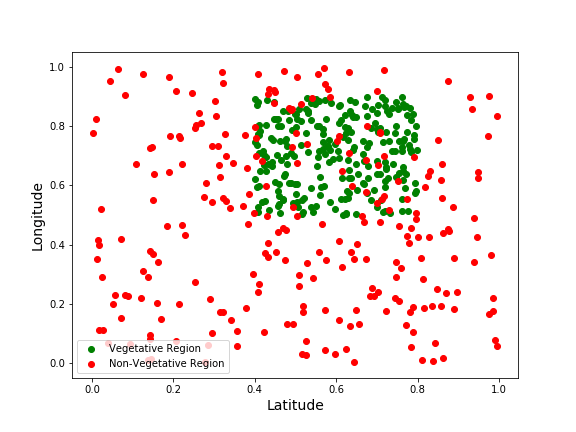A hand-labelled scatterplot. This example is inspired by CS109A, a course offered at Harvard College in the Fall of 2016.

One of the most intuitive ways to solve this problem is to merely look at the test point’s closest neighbors. Take, for example, a test point at (.35, .4), colored black below:

We want to predict whether this coordinate represents a vegetative or a non-vegetative region. To do this, we can select, say, the 3 nearest dots. These three dots are all labeled as non-vegetative, so we’ll classify the test point as non-vegetative as well

Now we know why this model contains the phrase nearest neighbors—because we classify a test point based on that point’s already-classified neighbors. What’s the K for? Simple: K is the number of neighbors we look at when determining how to classify a test point.

If K is really small (say K = 1), we’ll only look at the singly closest neighbor when classifying our test point. If K is really large (say K = 100), we’ll look at a huge range of neighbors before we make a classification.

The K value that you choose when making classifications depends entirely on the problem at hand and is often not easily intuited. Instead, data scientists will try plugging in many different values for K and seeing which value leads to the most accurate classifications.

Note that K is a parameter of the model, but it’s not necessarily a parameter that the model learns through training. Rather, it’s a special parameter that the data scientist explicitly sets herself. We call these parameters hyperparameters, and you should know that a data scientist often decides which hyperparameter to use by evaluating a bunch of different possibilities for that hyperparameter (say, K = 1, 5, 10, and 50). The process of evaluating these possible hyperparameters is called tuning.

Putting on the brakes: Why are hyperparameters important consideration for non-technical decision makers? It has to do with the way that most common software packages are implemented.

Most ML code libraries use default hyperparameters in the event that the data scientist forgets to explicitly set them herself. For example, if I forget to choose a value for K when running my KNN model in code, the software will assume I wanted K to be, say, 5. But the value of K is important and can have big implications for the results of the model.

Should software packages be allowed to use default hyperparameters? I’m not sure. Regardless, the important takeaway here is to always ask whether or not a model has been tuned properly — otherwise, it might not be doing its job correctly.

## Lastly, a (very) brief discussion about artificial neural nets…

It is not the goal of this post to describe in-detail how specific ML models work. That said, neural nets are especially important for non-technical readers because they’re incredibly powerful and are becoming ubiquitous in nearly any technology that merits the use of ML.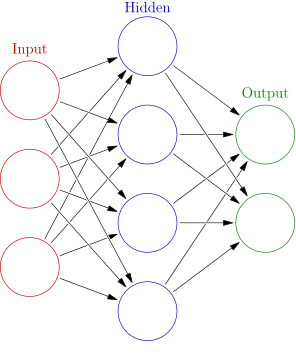https://en.wikipedia.org/wiki/Artificial_neural_network Borrowed from A toy neural net with 9 artificial neurons.

Neural nets are biologically inspired models, wherein collections of interconnected “neurons” (often called units or nodes) work together to transform input data to output data. Each node applies a simple mathematical transformation to the data it receives; it then passes its result to the other nodes in its path.

Just as our brain contains billions of biological neurons, neural nets typically contain thousands or millions of these artificial neurons.

Each connection between nodes represents a different parameter to the model. This means, of course, that neural nets with millions of nodes have potentially billions of parameters associated with them.

A common criticism of neural nets is that they are too difficult to interpret — that it’s too hard to understand “what’s going on” inside of them. This criticism springs from the fact that neural nets simply have too many parameters, most or all of which are determined via complex combinations of mathematical transformations to the training data. While a simple linear regression has just two parameters (m and b), a neural network can have infinitely more, and it can be hard to figure out how they were calculated.

Putting on the brakes: We often run into a trade off between a model’s interpretability and its predictive power. Neural nets are hugely powerful, but are almost impossible to interpret. On the other hand, simple linear regression is considerably easier to understand, but it’s generally not as powerful.

Should we trust the results of complicated neural nets if we can’t interpret their parameters? Why might it be a good idea to trust neural nets used in criminal justice situations to determine sentence length? Why might it be a bad idea?

The answers to these questions are critically important. As a general rule of thumb, machine learning models have to balance a tradeoff between being easy to understand and being powerful. Really powerful models like neural nets are often called black boxes because we’re not quite sure what’s inside of them, but these models can be extremely predictive.

When building models — or when telling others to build them as a CEO or president or professor might do—we must decide what balance we’d like to strike between building models that are predictive and building models that are easy to understand.

This balance almost always depends on the domain of the problem, of course. Having a neural net predict who we should let out of jail is probably a poor idea, given how hard it is to understand exactly why the neural net is making certain predictions. On the hand, using a simple linear regression is also inappropriate because its predictions will likely be poor.

## Key Takeaways:

What are the big concepts that you should take away from this post? You should know that…

1. Machine learning combines computer science and statistics to create statistical models, which are then used to make predictions about the world or to infer patterns in your data.
2. Statistical models are really just mathematical functions (e.g. Y = m × X + b). They are determined by their parameters (e.g. m and b), which are learned via the training process. Models also have hyperparameters (e.g. the K in KNN), which are tuned by trying out many possibilities.
3. ML models learn from training data, which captures our knowledge about the past.
4. There are, in general, two types of supervised models: those used for regression (like simple linear regression) and those used for classification (like KNN).
5. Regardless of whether or not you’re building these models yourself, there are a handful of important ethical questions that require deep thought before you take action on the results of an ML model.

## A parting message:

People tend to view much of ML as magic, and I hoped I showed you that this assumption is far from the truth. Assuming that models (like neural nets) are omniscient and infallible could potentially cause us to put too much faith in their output. Knowing how many different components go into building a model should open your eyes to the many opportunities for bias and error in a model’s output, which should be fiercely scrutinized before being trusted.

Join SafeGraph: We’re bringing together a world-class team, see open positions.

## Browse the latest